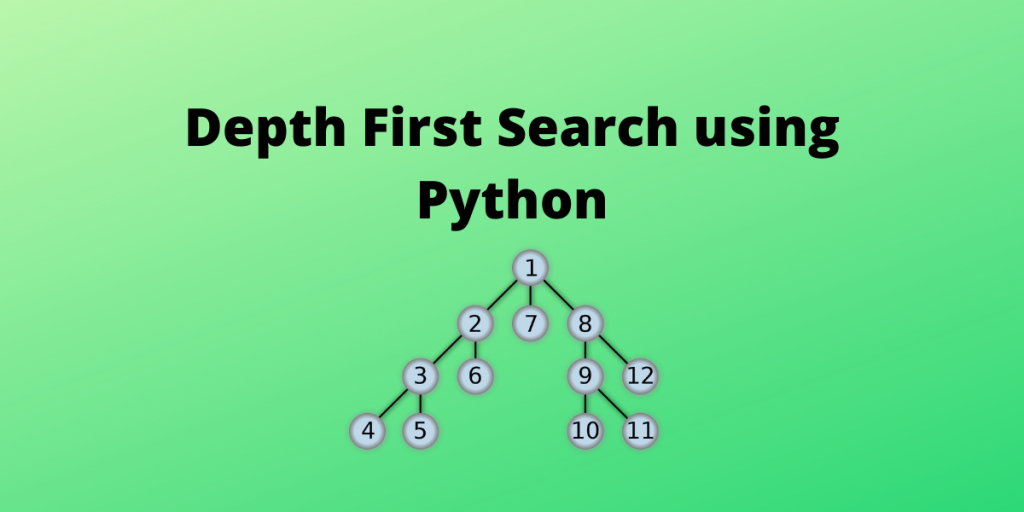# Depth First Search Algorithm using PythonDear readers, in this article I will walk you through the concept of Depth First Search (DFS). This is a graph concept which is a common problem in many competitive coding exams. So, let’s look at creating a DFS traversal using Python.

## What is Depth First Search?

The depth-first search is an algorithm that makes use of the Stack data structure to traverse graphs and trees. The concept of depth-first search comes from the word “depth”. The tree traverses till the depth of a branch and then back traverses to the rest of the nodes.

Consider an empty “Stack” that contains the visited nodes for each iteration. Our task here is as follows:

1. Start at the root node and push it onto the stack.
2. Check for any adjacent nodes of the tree and select one node.
3. Traverse the entire branch of the selected node and push all the nodes into the stack.
4. Upon reaching the end of a branch (no more adjacent nodes) ie nth leaf node, move back by a single step and look for adjacent nodes of the n-1th node.
5. If there are adjacent nodes for the n-1th node, traverse those branches and push nodes onto the stack.

## Concept of Depth First Search Illustrated

Let’s look into our example graph below:

A is the root node. So since A is visited, we push this onto the stack.

```Stack : A
```

Let’s go to the branch A-B. B is not visited, so we go to B and push B onto the stack.

```Stack : A B
```

Now, we have come to the end of our A-B branch and we move to the n-1th node which is A. We will now look at the adjacent node of A which is S. Visit S and push it onto the stack. Now you have to traverse the S-C-D branch, up to the depth ie upto D and mark S, C, D as visited.

```Stack: A B S C D
```

Since D has no other adjacent nodes, move back to C and traverse its adjacent branch E-H-G to the depth and push them onto the stack.

```Stack : A B S C D E H G
```

On reaching D, there is only one adjacent node ie F which is not visited. Push F onto the stack as well.

```Stack : A B S C D E H G F
```

This stack itself is the traversal of the DFS.

## Coding Depth First Search Algorithm in Python

As you must be aware, there are many methods of representing a graph which is the adjacency list and adjacency matrix.

So in the following example, I have defined an adjacency list for each of the nodes in our graph.

```graph1 = {
'A' : ['B','S'],
'B' : ['A'],
'C' : ['D','E','F','S'],
'D' : ['C'],
'E' : ['C','H'],
'F' : ['C','G'],
'G' : ['F','S'],
'H' : ['E','G'],
'S' : ['A','C','G']
}
```

Note: This adjacency list can be inputted from the user and need not be hard-coded.

Now, we will define our DFS function which takes in 3 parameters as input – the graph (adjacency list), a node, and a list of visited nodes.

If the current node is not visited ie not present in the visited list, mark it as visited and append it into the visited list.

Move to the next node and then recursively pass this node into the DFS function. This way each node moves till the depth and prints it as the DFS output.

```def dfs(graph, node, visited):
if node not in visited:
visited.append(node)
for k in graph[node]:
dfs(graph,k, visited)
return visited

visited = dfs(graph1,'A', [])
print(visited)
```

## Complete Code and Output

```graph1 = {
'A' : ['B','S'],
'B' : ['A'],
'C' : ['D','E','F','S'],
'D' : ['C'],
'E' : ['C','H'],
'F' : ['C','G'],
'G' : ['F','S'],
'H' : ['E','G'],
'S' : ['A','C','G']
}

def dfs(graph, node, visited):
if node not in visited:
visited.append(node)
for k in graph[node]:
dfs(graph,k, visited)
return visited

visited = dfs(graph1,'A', [])
print(visited)
```

The output of the above code is as follows:

```['A', 'B', 'S', 'C', 'D', 'E', 'H', 'G', 'F']
```

## Conclusion

I hope you have followed this tutorial on the DFS algorithm and have been able to understand the code and the example as well. Do try it out using pen and paper beside you to understand the traversals better.